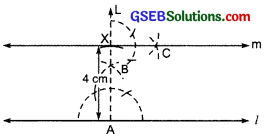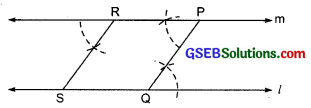# GSEB Solutions Class 7 Maths Chapter 10 Practical Geometry Ex 10.1

Gujarat Board GSEB Textbook Solutions Class 7 Maths Chapter 10 Practical Geometry Ex 10.1 Textbook Questions and Answers.

## Gujarat Board Textbook Solutions Class 7 Maths Chapter 10 Practical Geometry Ex 10.1Question 1.
Draw a line, say AB, take a point C outside it. Through C, draw a line parallel to AB using ruler and compasses only.
Solution:
Steps of construction:
I. Draw a line AB, and mark a point C outside it.
II. Mark another point D on AB and join CD.
III. With D as centre and a convenient radius, draw an arc cutting AB at E and CD at F.
IV. With C as centre and radius equal to DE draw an arc, cutting CD at G.
V With G as centre and radius (opening) equal to EF, mark a point H on the above arc.
VI. Join CH to draw a line ‘m’
Thus, ‘m’ is the required line such that m || AB.Question 2.
Draw a line l. Draw a perpendicular to l at any point on l. On this perpendicular choose a point X, 4 cm away from l. Through X, draw a line m parallel to l.
Solution:
Steps of construction:
I. Draw a line l and mark a point A on it.
II. Construct an angle of 90° at A to draw AL perpendicular to l.
III. Mark a point X on AL such that AX = 4 cm.
IV. At X, construct an angle of 90° to draw a perpendicular (m) to AL.
Thus, ‘m’ is the required line through X such that m || l.Question 3.
Let l be a line and P be a point not on l. Through P, draw a line m parallel to l. Now join P to any point Q on l. Choose any other point R on m. Through R, draw a line parallel to PQ. Let this meet l at S. What shape do the two sets of parallel lines enclose?
Solution:
Steps of construction:
I. Draw a line l and mark a point P outside it.
II. Take a point Q on l and join PQ.
III. Draw m || through P.
IV. Mark a point R on m.
V. Through R, draw RS || PQ, such that it meets l at S.
Since, l || m, so RP || SQ
Also PQ || RS
∴ PQRS is a parallelogram.